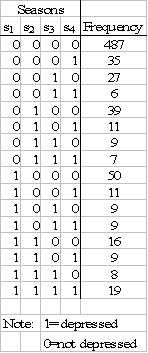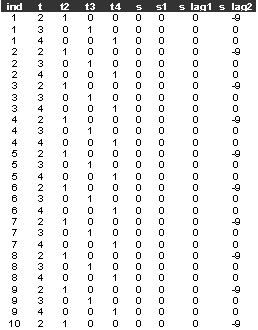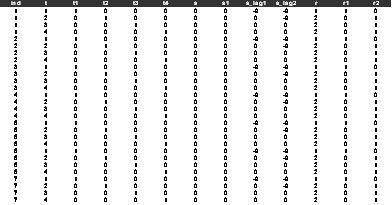Example FOL1. Depression in LA

The data in the table below were collected in a one year panel study of depression and help-seeking behaviour in Los Angeles (Morgan et al, 1983). Adults were interviewed during the spring and summer of 1979 and re-interviewed at 3 monthly intervals. A respondent was classified as depressed if they scored >16 on a 20 item list of symptoms.However, depression is difficult to overcome suggesting that state dependence might explain at least some of the observed temporal dependence, although it remains an empirical issue whether true contagion extends over three months.  We might also expect seasonal effects due to the weather.

References

Morgan, T.M., Aneshensel, C.S. & Clark, V.A. (1983), Parameter estimation for mover stayer models: analysis of depression over time, Soc Methods and Research, 11, 345-366.

Conditional analyses

(1) Conditional on the initial response

Data description (dep_rob2.dat):

The model for the binary depression data (dep_rob2.dat), ignoring the model for the initial response, has constant, dummy variables for seasons 3 and 4 and the lagged response variable.

The data (dep_rob2.dat) has:

Number of observations: 2256

Number of cases: 752

The variables are:

ind=individual identifier

t=season

t2=1 if t=2, 0 otherwise

t3=1 if t=3, 0 otherwise

t4=1 if t=4, 0 otherwise

s= binary indicator for depression (1 if yes, 0 otherwise)

s1=baseline response

s_lag1=lag 1 response

s_lag2=lag 2 response (not used)

The first few lines of the data set (dep_rob2.dat) look like:Sabre commands

out depression1.log

data ind t t2 t3 t4 s s1 s_lag1 s_lag2

read dep_rob2.dat

case ind

yvar s

link p

constant cons

mass 24

fit t3 t4 s_lag1 cons

dis m

dis e

stop

Sabre log file

Log likelihood =     -831.56731     on    2251 residual degrees of freedom

Parameter              Estimate         Std. Err.

___________________________________________________

cons                   -1.2942          0.72379E-01

t3                    -0.15466          0.88638E-01

t4                    -0.21480E-01      0.87270E-01

s_lag1                 0.94558          0.13563

scale                  0.32841          0.18226

The coefficient on   s_lag1 is 0.94558 (s.e. 0.13563), which is highly significant, but the scale parameter  is of marginal significance, suggesting a nearly homogeneous first order model. Can we trust this inference?

(2) Condition on the initial response but allow the random effect to be dependent on initial response and time constant covariates, Wooldridge (2005)

The model for the binary depression data (dep_rob2.dat), ignoring the model for the initial response, has constant, dummy variables for seasons 3 and 4, the lagged response variable and the initial response.

References

Wooldridge, J.M., (2005), Simple solutions to the initial conditions problem in dynamic, nonlinear panel data models with unobserved heterogeneity, Journal of Applied Econometrics, 20, 39---54.

Sabre commands

out depression1b.log

data ind t t2 t3 t4 s s1 s_lag1 s_lag2

read dep_rob2.dat

case ind

yvar s

link p

constant cons

mass 24

fit t3 t4 s_lag1 s1 cons

dis m

dis e

stop

Gives

Log likelihood =     -794.75310     on    2250 residual degrees of freedom

Parameter              Estimate         Std. Err.

___________________________________________________

cons                   -1.6646          0.11654

t3                    -0.20988          0.99663E-01

t4                    -0.88079E-01      0.97569E-01

s_lag1                 0.43759E-01      0.15898

s1                      1.2873          0.19087

scale                  0.88018          0.12553

This has the lagged response s_lag1 estimate at 0.43759E-01 (0.15898), which is not significant, while the initial response s1 estimate 1.2873 (0.19087) and the scale parameter estimate 0.88018 (0.12553) are highly significant. There is also a big improvement in the log-likelihood over the model without s1 of  73.63   for 1 df. This model has no time-constant covariates to be confounded by the auxiliary model and suggests that depression is a zero order process.

Modelling the initial conditions (joint analysis)

There are several approximations that can be adopted: (1) use the same random effect in the initial and subsequent responses, e.g. Crouchley and Davies (2001); (2) use a one-factor decomposition for the initial and subsequent responses, e.g. Heckman (1981a), Stewart (2007); (3) use different (but correlated) random effects for the initial and subsequent responses; (4) embed the Wooldridge (2005) approach in joint models for the initial and subsequent responses.

References

Crouchley, R., & Davies, R.B., (2001), A comparison of GEE & random effects models for distinguishing hetero-geneity, nonstationarity & state dependence in a collection of short binary event series, Stat Mod, 1, 271-285.

Heckman J.J., (1981a), Statistical models for discrete panel data, In Manski, C.F. & McFadden, D, (eds), Structural analysis of discrete data with econometric applications, MIT press, Cambridge, Mass.

Stewart, M.B., (2007), The interrelated dynamics of unemployment and low-wage employment

Journal of Applied Econometrics, Volume 22, 511-531

Wooldridge, J.M., (2005), Simple solutions to the initial conditions problem in dynamic, nonlinear panel data models with unobserved heterogeneity, Journal of Applied Econometrics, 20, 39---54.

(1) Same random effect in the initial and subsequent responses with a common scale parameter

The joint model for the binary depression data (depression.dat) has a constant for the initial response, a constant for the subsequent responses, dummy variables for seasons 3 and 4 and the lagged response variable.

Data description

Number of observations: 3008

Number of cases: 752

The variables are:

ind=individual identifier

t=season (1,2,3,4)

t1=1 if t=1, 0 otherwise

t2=1 if t=2, 0 otherwise

t3=1 if t=3, 0 otherwise

t4=1 if t=4, 0 otherwise

s= binary indicator for depression (1 if yes, 0 otherwise)

s1=baseline response

s_lag1=lag 1 response, -9 if missing

s_lag2=lag 2 response, -9 if missing (not used)

r=response position, 1 if baseline, 2 if subsequent response

r1=1 if r=1, 0 otherwise

r2=1 if r=2, 0 otherwise

The first few lines of the data set (depression.dat) look like:In the depression example, the model for the initial response (indicator r1=1), has only a constant, the model for the 3 subsequent responses (indicator r2=1) has a constant, dummy variables for seasons 3 (r2_t3 ) and 4 (r2_t4), and the lagged response variable(r2_lag1).

Sabre commands

out depression2.log

data ind t t1 t2 t3 t4 s s1 s_lag1 s_lag2 r r1 r2

read depression.dat

case ind

yvar s

link p

trans r2_t3 r2 * t3

trans r2_t4 r2 * t4

trans r2_lag1 r2 * s_lag1

mass 24

fit r1 r2 r2_t3 r2_t4 r2_lag1

dis m

dis e

stop

Gives

Log likelihood =     -1142.9749     on    3002 residual degrees of freedom

Parameter              Estimate         Std. Err.

___________________________________________________

r1                     -1.3476          0.10026

r2                     -1.4708          0.92548E-01

r2_t3                 -0.20740          0.99001E-01

r2_t4                 -0.85438E-01      0.97129E-01

r2_lag1                0.70228E-01      0.14048

scale                   1.0372          0.10552

The coefficient of r2_lag1 is 0.70228E-01 (0.14048) suggesting that there is no state dependence in these data, while the scale coefficient 1.0372 (0.10552) suggests heterogeneity.

(2) Same random effect in the initial and subsequent responses but with different scale parameters

As in the common scale parameter model, the joint model for the binary depression data (depression.dat) has a constant for the initial response, a constant for the subsequent responses, dummy variables for seasons 3 and 4 and the lagged response variable.

Sabre commands

out depression3.log

data ind t t1 t2 t3 t4 s s1 s_lag1 s_lag2 r r1 r2

read depression.dat

case ind

yvar s

rvar r

link p

trans r2_t3 r2 * t3

trans r2_t4 r2 * t4

trans r2_lag1 r2 * s_lag1

mass 24

depend y

nvar 1

fit r1 r2 r2_t3 r2_t4 r2_lag1

dis m

dis e

stop

Gives

Log likelihood =     -1142.9355     on    3001 residual degrees of freedom

Parameter              Estimate         Std. Err.

___________________________________________________

r1                     -1.3248          0.12492

r2                     -1.4846          0.10639

r2_t3                 -0.21020          0.10004

r2_t4                 -0.87882E-01      0.98018E-01

r2_lag1                0.50254E-01      0.15792

scale1                  1.0021          0.15927

scale2                  1.0652          0.14587

This shows that the state dependence regressor r2_lag1 has estimate 0.50254E-01 (0.15792), which is not significant. It also shows that the scale parameters are nearly the same. The log likelihood improvement of the model with 2 scale parameters over that of the previous model with 1 scale parameter is  0.079 for 1 df. Thus the model with 1 scale parameter is to be preferred.

(3) Different random effects in the initial and subsequent responses

As in the single random effect models, the joint model for the binary depression data (depression.dat) has a constant for the initial response, a constant for the subsequent responses, dummy variables for seasons 3 and 4 and the lagged response variable.

Sabre commands

out depression4.log

data ind t t1 t2 t3 t4 s s1 s_lag1 s_lag2 r r1 r2

data ind t t1 t2 t3 t4 s s1 s_lag1 s_lag2 r r1 r2

read depression.dat

case ind

yvar s

model b

rvar r

link first=p second=p

trans r2_t3 r2 * t3

trans r2_t4 r2 * t4

trans r2_lag1 r2 * s_lag1

mass first=24 second=24

eqscale y

der1 y

nvar 1

fit r1 r2 r2_t3 r2_t4 r2_lag1

dis m

dis e

stop

Gives

Log likelihood =     -1142.9355     on    3001 residual degrees of freedom

Parameter              Estimate         Std. Err.

___________________________________________________

r1                     -1.3672          0.12386

r2                     -1.4846          0.10591

r2_t3                 -0.21020          0.10033

r2_t4                 -0.87881E-01      0.97890E-01

r2_lag1                0.50253E-01      0.15946

scale                   1.0652          0.14362

corr                   0.97091          0.10087

Note that the log likelihood is exactly the same as for the previous model, the scale2 parameter from the previous model has the same value as the scale parameter of the current model. The lagged response r2_lag1 has an estimate of 0.50313E-01 (0.15945), which is not significant. The correlation between the random effects (corr) has estimate 0.97089 (0.10093), which is very close to 1 suggesting that the common random effects, zero order, single scale parameter model is to be preferred.

(4) Embed the Wooldridge (2005) approach in joint models for the initial and subsequent responses

As in the single random effect models, the joint model for the binary depression data (depression.dat) has a constant for the initial response, a constant for the subsequent responses, dummy variables for seasons 3 and 4, the lagged response variable and the initial response variable.

Sabre commands

out depression5.log

data ind t t1 t2 t3 t4 s s1 s_lag1 s_lag2 r r1 r2

read depression.dat

case ind

yvar s

link p

trans r2_t3 r2 * t3

trans r2_t4 r2 * t4

trans r2_lag1 r2 * s_lag1

trans r2_base r2 * s1

mass 24

fit r1 r2 r2_t3 r2_t4 r2_lag1 r2_base

dis m

dis e

stop

Gives

Log likelihood =     -1142.9670     on    3001 residual degrees of freedom

Parameter              Estimate         Std. Err.

___________________________________________________

r1                     -1.3632          0.16189

r2                     -1.4741          0.97129E-01

r2_t3                 -0.20869          0.99797E-01

r2_t4                 -0.86541E-01      0.97774E-01

r2_lag1                0.61490E-01      0.15683

r2_base               -0.33544E-01      0.26899

scale                   1.0602          0.21274

This joint model has both the lagged response r2_lag1 estimate of 0.61490E-01 (0.15683) and the baseline/initial response effect r2_base estimate of -0.33544E-01 (0.26899) as being non-significant.

If we estimate a standard zero order model with dummy variables for seasons 2, 3, and 4 to all the data we get

Parameter              Estimate         Std. Err.

___________________________________________________

cons                   -1.3712          0.90331E-01

t2                    -0.10411          0.94043E-01

t3                    -0.31491          0.98105E-01

t4                    -0.19507          0.95630E-01

scale                   1.0734          0.78059E-01

This model, without any state dependence, suggests that the worst seasons are t3 (autumn) and t4 (winter). This model also has a good fit to the data, the log L(Data)=-1141.54, so the ChiSq (goodness of fit to the data) is  3.11 with 10 df.